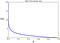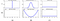# Shannon entropy in the context of machine learning and AI

In this post, I want to elaborate on the concept of Shannon entropy in the context machine learning and AI. My goal is to provide some insight into the math behind Shannon entropy, but keep the discussion relatively informal.

So let’s dive right in. It is common in machine learning to quantify the expected amount of information associated with stochastic events, and to quantify the similarity between probability distributions. In both cases, Shannon entropy is used as a measure for information content of probability distributions. It is named after the father of information theory, Claude Shannon (1916–2001).

## Self-information

The fundamental concept behind Shannon entropy is the so-called self-information of an event, sometimes called surprisal. The intuition behind self-information is as follows. When an unlikely outcome of an event (random variable) is observed, we associate it with a high amount of information. Contrarily, when a more likely outcome is observed, we associate it with a smaller amount of information. It is very helpful to think of self-information as the surprise associated with an event. Consider, for example, a heavily biased coin that always lands on heads. The outcome of any coin toss is fully predictable, thus we will never be surprised about the outcome, which means that we gain zero information from such an experiment. In other words, its self-information is zero. If the coin is biased less heavily, there is some amount of surprise each time we toss the coin, although more than 50% of the time we will still see heads. Consequently, the self-information is larger than zero. If the coin is biased towards always landing on tails (instead of heads), we again end up with zero entropy, or surprise. The maximum amount of surprise is obtained in the case of an unbiased coin where the chances of landing on heads or tails are both 50%, as this is the situation where the outcome of the coin toss is least predictable.

Based on the above informal requirements, we can find an appropriate function to describe self-information. For a discrete random variable X with possible values x_1, . . . , x_n and probability mass function P(X), any positive and monotonically decreasing function I(p_i) between 0 and 1 could be used as a measure for information. So far so good. One additional and crucial property, however, is additivity of independent events; the self-information of two subsequent coin tosses should be twice the self-information of a single coin toss. This makes sense for independent variables, as the amount of surprise, or unpredictability, becomes twice larger in this case. Formally, we need I(p_i·p_j) = I(p_i)+I(p_j) for independent events x_i and x_j. The function fulfilling all of these requirements is the negative logarithm,

Figure 1 shows a plot of I(p).Figure 1. The self-information function I(p). Low probabilities are associated with high self-information, and vice versa.

Let’s go back to our simple coin toss experiment. In the language of information theory, one bit (also called ”Shannon”) of information is required to represent the two possible outcomes of a single coin toss. Similarly, for two consecutive coin tosses, two bits of information are required to describe all four possible outcomes. In general, log_2(n) bits of information are required to describe the outcome, or equivalently the self-information, of n subsequent independent random events. Let’s walk through the simple calculations for three subsequent coin tosses. There are 2^3 = 8 possible outcomes, and the probability of any particular outcome is 0.5^3 = 0.125. Therefore, the self-information of this experiment is I(0.125) = −log_2(0.125) = 3. We need 3 bits to represent all possible outcomes, so the self-information of any particular sequence of three coin tosses equals 3.0.

We can also calculate the self-information of a continuous random variable. Figure 2 shows three different pdfs and their corresponding information content. The Dirac delta in 2a corresponds to the strong bias, zero entropy case of a biased coin that always lands on the same side. An infinitely high information content would be associated wherever p(x) = 0. However, this is hypothetical since these events will never actually occur due to zero probability. The Gaussian pdf in Figure 2B is analogous to the biased coin that tends to fall on the same side often, but not always. Lastly, Figure 2C depicts a uniform pdf, with associated uniform information content, analogous to our unbiased coin toss.Figure 2. Three different probability densities p on [−3,+3] and their self-information I(p). (A) Dirac delta function (completely deterministic) (B) Gaussian with μ = 0,σ = 0.5 (biased towards x = 0) (C.) Uniform distribution.

## Entropy

So far we have only discussed self-information. For the case of a fair coin, self-information actually equals Shannon entropy, because all outcomes are equal in probability. In general, however, Shannon entropy is the average self-information (expected value) over all possible values of X,

where b is the base of the logarithm. Above we used b = 2, other common choices are b = 10 as well as Euler’s number e, but the choice doesn’t matter much, since logarithms of varying bases are related by a constant. We will assume base 2 from here on and omit b.

If you paid close attention, you may wonder what happens to entropy when p(x_i) = 0, since in this case we have to compute 0 · log(0). It turns out that
lim p→0 p · log(p) = 0. A mathematical proof can be established using the rule of L’Hospital.

Shannon entropy generalizes to the continuous domain, where it is referred to as differential entropy (some restrictions apply which shall not be discussed here). For a continuous random variable x and probability density function p(x), Shannon entropy is defined as follows,

The entropy for our three example distributions is 0 (Dirac delta), 174 (Gaussian), and 431 (uniform). The pattern that emerges from our experiment is that broad distributions have the highest entropy. It’s important for your understanding to take a closer look at Figure 2B and 2C. Despite the fact that in the Gaussian case the area under I(p) is much larger than in the uniform case, the entropy is much smaller than in the uniform case because I(p) is weighted by the probability density function p, which is close to zero on both sides of the Gaussian. The best analogy to keep in mind to remember that a broad probability density has high entropy is to imagine a gas filled in a tank. We know from physics that the entropy in a closed system increases over time, and never decreases on its own. The distribution of gas particles (number of gas particles per unit volume) converges to a uniform value across the tank, after we infuse the gas on either side of the tank. Low entropy would mean that a higher density gas particles accumulated in certain areas, which never happens on its own. Accumulation of many gas particles in a small region corresponds to our Gaussian pdf, and in an extreme case to the Dirac delta function, when all gas particles are condensed into an infinitely small region.

## Cross entropy

Cross entropy is a mathematical tool for comparing two probability distributions p and q. It is similar to entropy, but instead of the expectation of log(p) under p, we compute the expectation of log(q) under p,

In the language of information theory, this quantity gives us the average number of bits required to code an event from q if we use the ”wrong” coding scheme q instead of p. In machine learning, it is a very useful measure for the similarity of probability distributions and serves as a loss function (more details below).

# Uses in machine learning

You may wonder, at this point, how entropy is relevant in machine learning. So let’s look at a some specific areas next.

## Bayesian learning

First, the Gaussian case described above is important, as the normal distribution is a very common modeling choice in machine learning applications. The goal in machine learning is to reduce entropy. We want to make predictions, and we have to be confident about our predictions. Entropy gives us a measure to quantify this confidence. In a Bayesian setting, often a prior distribution is assumed that has a broad pdf, reflecting the uncertainty in the value of the random variable before an observation is made. When data comes in, the entropy reduces and causes the posterior to form peaks around the likely values of the parameters.

## Decision tree learning

In decision tree learning, entropy is used to build the tree. Constructing a decision tree starts at the root node, by splitting the data set S into a number of subsets according to all possible values of the ”best” attribute, i.e., the one that minimizes the (combined) entropy in the resulting subsets. This procedure is repeated recursively until there are no more attributes left to split. This prodecude is called ID3 algorithm.

## Classification

Cross entropy is the basis of the standard loss function for logistic regression and neural networks, in both binomial and multinomial classification scenarios. Usually, p is used for the true (or empirical) distribution (i.e., the distribution of the training set), and q is the distribution described by a model. Let’s look at binary logistic regression as an example. The two classes are labeled 0 and 1, and the logistic model assigns the probabilities q_(y=1) = ŷ and q_(y=0) = 1 − ŷ to each input x. This can be concisely written as q ∈ {ŷ, 1 − ŷ}. Even though the empirical labels p are always exactly 0 or 1, the same notation is used here, p ∈ {y, 1 − y}, so don’t get confused. Using this notation, the cross entropy between empirical and estimated distribution for a single sample is

When used as a loss function, the average of all cross entropies from all N samples is used,

## KL divergence

Closely related to cross entropy, the KL divergence from q to p, written DKL(p||q), is another similarity measure often used in machine learning. In the language of Bayesian Inference, DKL(p||q) is a measure of the information gained when one revises one’s beliefs from the prior distribution q to the posterior distribution p, or in other words, the amount of information lost when q is used to approximate p. It is used, for example, in training the latent space representation of a variational autoencoder. KL divergence can be expressed using entropy and cross entropy,

While cross entropy measures the average total bits needed to code an event from p when a coding scheme optimized for q is used, while the KL divergence gives the number of additional bits needed when the optimal coding scheme for q is used, instead of the optimal coding scheme for p. From this we can see that in the context of machine learning, where p is fixed, cross entropy and KL divergence are related by a constant additive term, so for the purpose of optimization they are equivalent. It may still make sense to talk about KL divergence rather than cross entropy from a theoretical perspective, and one useful property of KL divergence is that it is zero when p and q are equal.

# Conclusion

The purpose of this post is to shed some light on the concept of entropy in the context of machine learning and artificial intelligence, by explaining the most essential aspects of the theory, and where it appears in applications. The Python code for generating all results presented above can be found on this Gist.

Written by